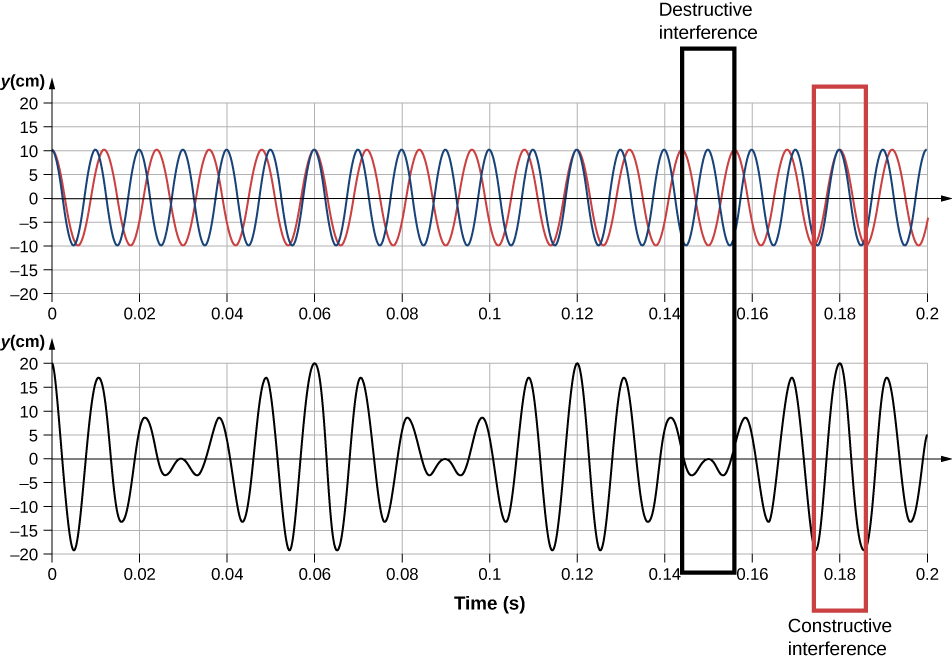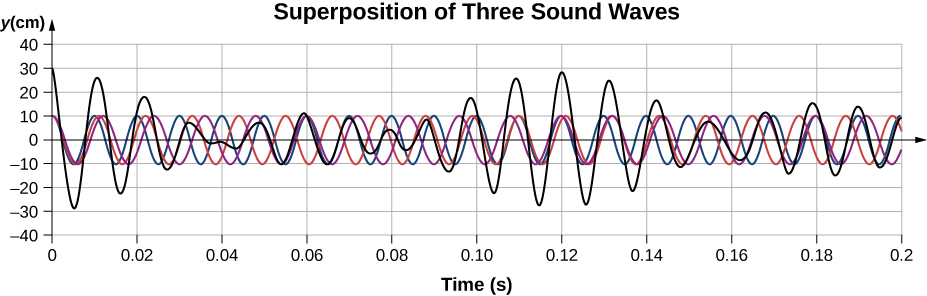17 Sound

# 17.6 Beats

### Learning Objectives

By the end of this section, you will be able to:

• Determine the beat frequency produced by two sound waves that differ in frequency
• Describe how beats are produced by musical instruments

The study of music provides many examples of the superposition of waves and the constructive and destructive interference that occurs. Very few examples of music being performed consist of a single source playing a single frequency for an extended period of time. You will probably agree that a single frequency of sound for an extended period might be boring to the point of irritation, similar to the unwanted drone of an aircraft engine or a loud fan. Music is pleasant and interesting due to mixing the changing frequencies of various instruments and voices.

An interesting phenomenon that occurs due to the constructive and destructive interference of two or more frequencies of sound is the phenomenon of beats. If two sounds differ in frequencies, the sound waves can be modeled as

${y}_{1}=A\,\text{cos}({k}_{1}x-2\pi {f}_{1}t)\,\text{and}\,{y}_{2}=A\,\text{cos}({k}_{2}x-2\pi {f}_{2}t).$

Using the trigonometric identity $\text{cos}\,u+\text{cos}\,v=2\,\text{cos}(\frac{u+v}{2})\text{cos}(\frac{u-v}{2})$ and considering the point in space as $x=0.0\,\text{m,}$ we find the resulting sound at a point in space, from the superposition of the two sound waves, is equal to Figure:

$y(t)=2A\,\text{cos}(2\pi {f}_{avg}t)\text{cos}(2\pi (\frac{|{f}_{2}-{f}_{1}|}{2})t),$

where the beat frequency is

${f}_{\text{beat}}=|{f}_{2}-{f}_{1}|.$Figure 17.29 Beats produced by the constructive and destructive interference of two sound waves that differ in frequency.

These beats can be used by piano tuners to tune a piano. A tuning fork is struck and a note is played on the piano. As the piano tuner tunes the string, the beats have a lower frequency as the frequency of the note played approaches the frequency of the tuning fork.

### Example

#### Find the Beat Frequency Between Two Tuning Forks

What is the beat frequency produced when a tuning fork of a frequency of 256 Hz and a tuning fork of a frequency of 512 Hz are struck simultaneously?

#### Strategy

The beat frequency is the difference of the two frequencies.

#### Solution

We use ${f}_{\text{beat}}=|{f}_{2}-{f}_{1}|:$

$|{f}_{2}-{f}_{1}|=(512-256)\,\text{Hz}=256\,\text{Hz}\text{.}$

#### Significance

The beat frequency is the absolute value of the difference between the two frequencies. A negative frequency would not make sense.

What would happen if more than two frequencies interacted? Consider three frequencies.

Show Solution

An easy way to understand this event is to use a graph, as shown below. It appears that beats are produced, but with a more complex pattern of interference.The study of the superposition of various waves has many interesting applications beyond the study of sound. In later chapters, we will discuss the wave properties of particles. The particles can be modeled as a “wave packet” that results from the superposition of various waves, where the particle moves at the “group velocity” of the wave packet.

### Summary

• When two sound waves that differ in frequency interfere, beats are created with a beat frequency that is equal to the absolute value of the difference in the frequencies.

### Conceptual Questions

Two speakers are attached to variable-frequency signal generator. Speaker A produces a constant-frequency sound wave of 1.00 kHz, and speaker B produces a tone of 1.10 kHz. The beat frequency is 0.10 kHz. If the frequency of each speaker is doubled, what is the beat frequency produced?

The label has been scratched off a tuning fork and you need to know its frequency. From its size, you suspect that it is somewhere around 250 Hz. You find a 250-Hz tuning fork and a 270-Hz tuning fork. When you strike the 250-Hz fork and the fork of unknown frequency, a beat frequency of 5 Hz is produced. When you strike the unknown with the 270-Hz fork, the beat frequency is 15 Hz. What is the unknown frequency? Could you have deduced the frequency using just the 250-Hz fork?

The frequency of the unknown fork is 255 Hz. No, if only the 250 Hz fork is used, listening to the beat frequency could only limit the possible frequencies to 245 Hz or 255 Hz.

Referring to the preceding question, if you had only the 250-Hz fork, could you come up with a solution to the problem of finding the unknown frequency?

A “showy” custom-built car has two brass horns that are supposed to produce the same frequency but actually emit 263.8 and 264.5 Hz. What beat frequency is produced?

Show Solution

The beat frequency is 0.7 Hz.

### Problems

What beat frequencies are present: (a) If the musical notes A and C are played together (frequencies of 220 and 264 Hz)? (b) If D and F are played together (frequencies of 297 and 352 Hz)? (c) If all four are played together?

What beat frequencies result if a piano hammer hits three strings that emit frequencies of 127.8, 128.1, and 128.3 Hz?

$\begin{array}{cc} \hfill {f}_{\text{B}}& =\hfill & |{f}_{1}-{f}_{2}|\hfill \\ \hfill |128.3\,\text{Hz}-128.1\,\text{Hz}|& =\hfill & 0.2\,\text{Hz;}\hfill \\ \hfill |128.3\,\text{Hz}-127.8\,\text{Hz}|& =\hfill & 0.5\,\text{Hz;}\hfill \\ \hfill |128.1\,\text{Hz}-127.8\,\text{Hz}|& =\hfill & 0.3\,\text{Hz}\hfill \end{array}$

A piano tuner hears a beat every 2.00 s when listening to a 264.0-Hz tuning fork and a single piano string. What are the two possible frequencies of the string?

Two identical strings, of identical lengths of 2.00 m and linear mass density of $\mu =0.0065\,\text{kg/m,}$ are fixed on both ends. String A is under a tension of 120.00 N. String B is under a tension of 130.00 N. They are each plucked and produce sound at the $n=10$ mode. What is the beat frequency?

$\begin{array}{cc} {v}_{A}=135.87\,\text{m/s,}\enspace{v}_{B}=141.42\,\text{m/s,}\hfill \\ {\lambda }_{A}={\lambda }_{B}=0.40\,\text{m}\hfill \\ \Delta f=15.00\,\text{Hz}\hfill \end{array}$

A piano tuner uses a 512-Hz tuning fork to tune a piano. He strikes the fork and hits a key on the piano and hears a beat frequency of 5 Hz. He tightens the string of the piano, and repeats the procedure. Once again he hears a beat frequency of 5 Hz. What happened?

A string with a linear mass density of $\mu =0.0062\,\text{kg/m}$ is stretched between two posts 1.30 m apart. The tension in the string is 150.00 N. The string oscillates and produces a sound wave. A 1024-Hz tuning fork is struck and the beat frequency between the two sources is 52.83 Hz. What are the possible frequency and wavelength of the wave on the string?

$\begin{array}{cc} v=155.54\,\text{m/s,}\hfill \\ {f}_{\text{string}}=971.17\,\text{Hz,}n=16.23\hfill \\ {f}_{\text{string}}=1076.83\,\text{Hz,}n=18.00\hfill \end{array}$ The frequency is 1076.83 Hz and the wavelength is 0.14 m.

A car has two horns, one emitting a frequency of 199 Hz and the other emitting a frequency of 203 Hz. What beat frequency do they produce?

The middle C hammer of a piano hits two strings, producing beats of 1.50 Hz. One of the strings is tuned to 260.00 Hz. What frequencies could the other string have?

Show Solution

$\begin{array}{cc} {f}_{2}={f}_{1}\pm{f}_{\text{B}}=260.00\,\text{Hz}\pm1.50\,\text{Hz,}\hfill \\ \text{so that}\,{f}_{2}=261.50\,\text{Hz}\,\text{or}\,{f}_{2}=258.50\,\text{Hz}\hfill \end{array}$

Two tuning forks having frequencies of 460 and 464 Hz are struck simultaneously. What average frequency will you hear, and what will the beat frequency be?

Twin jet engines on an airplane are producing an average sound frequency of 4100 Hz with a beat frequency of 0.500 Hz. What are their individual frequencies?

Show Solution

$\begin{array}{cc} {f}_{\text{ace}}=\frac{{f}_{1}+{f}_{2}}{2};{f}_{\text{B}}={f}_{1}-{f}_{2}(\text{assume}{f}_{1} \gt {f}_{2})\hfill \\ {f}_{\text{ace}}=\frac{({f}_{\text{B}}+{f}_{2})+{f}_{2}}{2}\Rightarrow \hfill \\ {f}_{2}=4099.750\,\text{Hz}\hfill \\ {f}_{1}=4100.250\,\text{Hz}\hfill \end{array}$

Three adjacent keys on a piano (F, F-sharp, and G) are struck simultaneously, producing frequencies of 349, 370, and 392 Hz. What beat frequencies are produced by this discordant combination?

### Glossary

beat frequency
frequency of beats produced by sound waves that differ in frequency
beats
constructive and destructive interference of two or more frequencies of sound